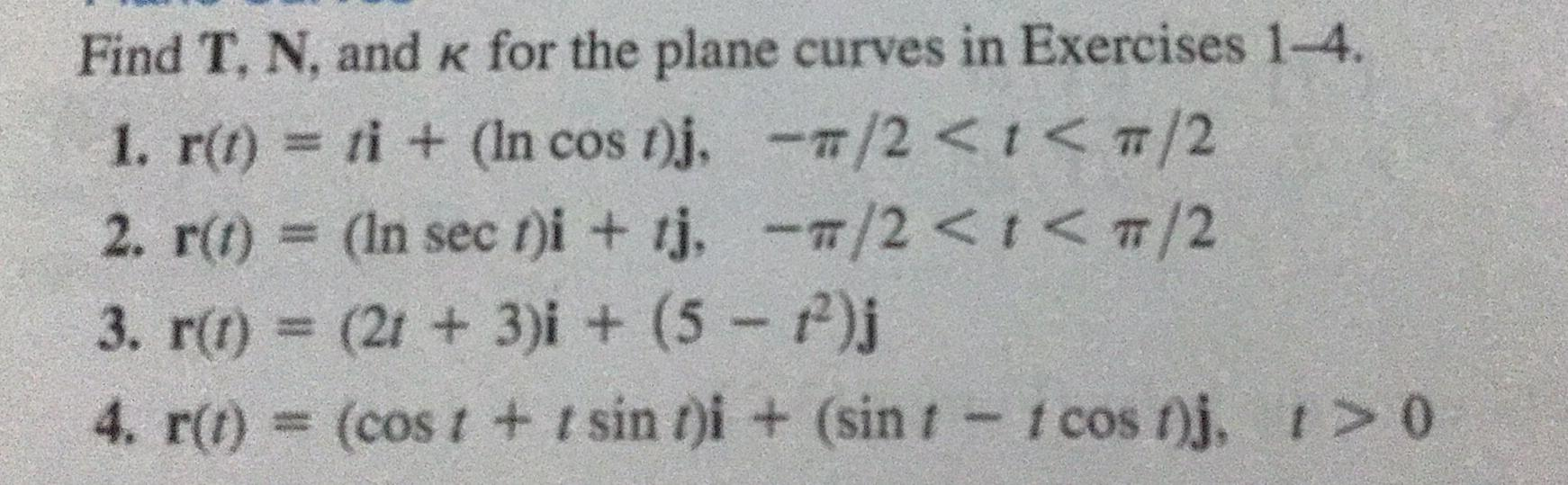Question:

# Find T, N, and K for the plane curves in Exercises 1-4. 1.

Last updated: 7/27/2022Find T, N, and K for the plane curves in Exercises 1-4. 1. r(t) = ti + (In cos t)j,-π/2 < t< π/2 2. r(t) = (In sect)i + tj,-π/2 < t< π/2 3. r(t) = (2t+ 3)i + (5 −t²)j 4. r(t) = (cost + t sin t)i + (sin 1 - 1 cost)j, t> 0